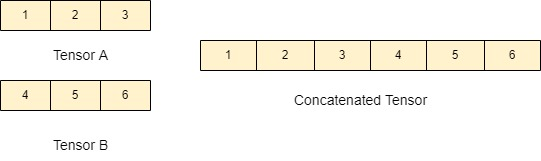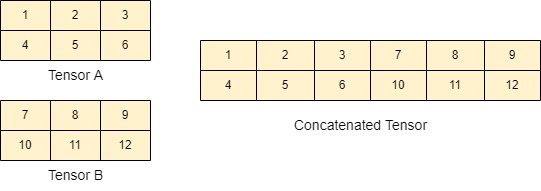# Concatenate Two Tensors Horizontally in TensorFlow

This quick article will explain the horizontal concatenation of two tensors using the `TensorFlow v2.8.2` framework in Python. First, we will discuss the visual representation of horizontal concatenation of the tensors in Python, and then we will see methods to concatenate them.

## Horizontal Concatenation of Tensors

Tensors are multi-dimensional arrays with a uniform type (called a `dtype`). There are many supported libraries in Python for multi-dimensional arrays (e.g., `NumPy`, `PyTorch`, `TensorFlow`, etc.).

The `TensorFlow` library is developed by Google and is one of the advanced and multifunctional libraries; best suited for creating and manipulating the tensors easily while performing machine learning tasks.

## Visual Representation of Tensors

A tensor can be multiple dimensional. For example: 1-dimensional (Rank-1 tensor), 2-dimensional (Rank-2 tensor), 3-dimensional (Rank-3 tensor) and so on.

### One-dimensional Tensors Horizontal Concatenation

For horizontal concatenation, the tensors must have the same first dimension. In the below diagram, the horizontal concatenation of Tensor A (`shape=(1,3)`) and Tensor B (`shape=(1,3)`) gives the resultant tensor.

In this case, Tensor A and Tensor B have the same first dimension (i.e., equal to 1). The concatenated tensor will be of `shape=(1, 6)` as depicted in the following diagram.### Two-dimensional Tensors Horizontal Concatenation

A 2-dimensional tensor looks like a matrix. In the below diagram, the horizontal concatenation of two 2-dimensional Tensors (i.e., Tensor A (`shape=(2,3)`) and Tensor B (`shape=(2,3)`)) gives the resultant tensor.

Note that Tensor A and Tensor B have the same first dimension (i.e., equal to 2), and the resultant concatenated tensor is of `shape=(2, 6)`.## Concatenate Two Tensors Horizontally in TensorFlow

Now, we will see how we can concatenate the tensors horizontally by using `TensorFlow` in Python.

``````pip install tensorflow
``````

First, use the above command to install the `TensorFlow` library.

``````import tensorflow as tf
t1 = [[1, 2], [3, 4]]
t2 = [[5, 6, 7], [8, 9, 10]]
result=tf.concat([t1, t2], 1)
print(result)
``````

In the above code, we first imported the `TensorFlow` library and then declared two tensors `t1` and `t2` of shapes `(2, 2)` and `(2, 3)` respectively. After that, we used the `tf.concat(values, axis, name)` function to concatenate the tensors along axis 1.

Here, the `axis= 1` means that the tensors will be concatenated horizontally.

The `tf.concat(values, axis, name)` function takes the following three arguments.

1. `values` - list of tensors.
2. `axis` - A 0-dimensional tensor that represents the dimension to concatenate.
3. `name` (optional) - Represents the operation’s name.

A detailed description of the `concat` function can be found here.

The above code gives the following output tensor.

``````tf.Tensor(
[[ 1  2  5  6  7]
[ 3  4  8  9 10]], shape=(2, 5), dtype=int32)
]
``````

The resultant tensor is of shape `(2, 5)`. This is evident because the `result` tensor comprises 2 rows and 5 columns.

Write for us
DelftStack articles are written by software geeks like you. If you also would like to contribute to DelftStack by writing paid articles, you can check the write for us page.